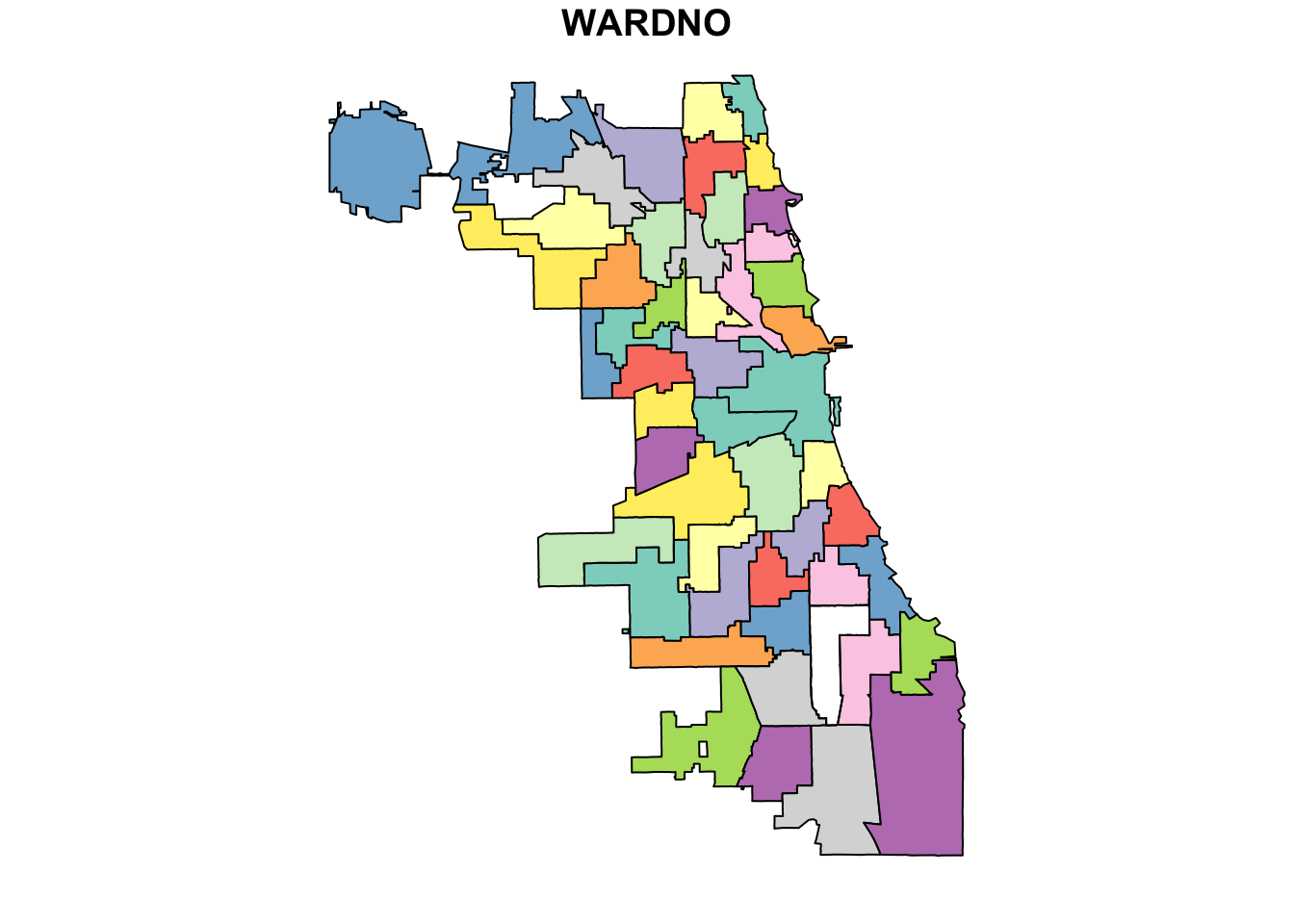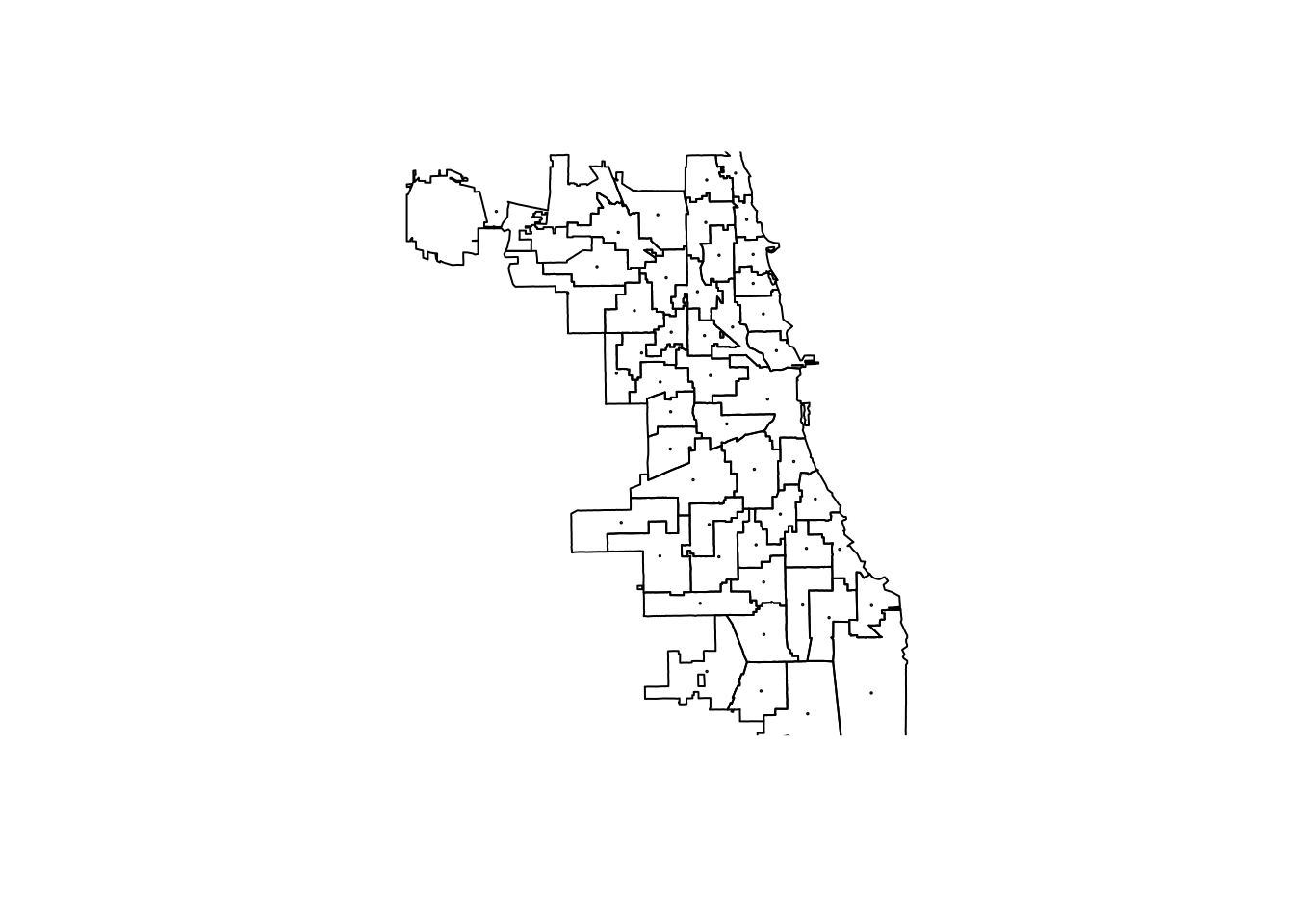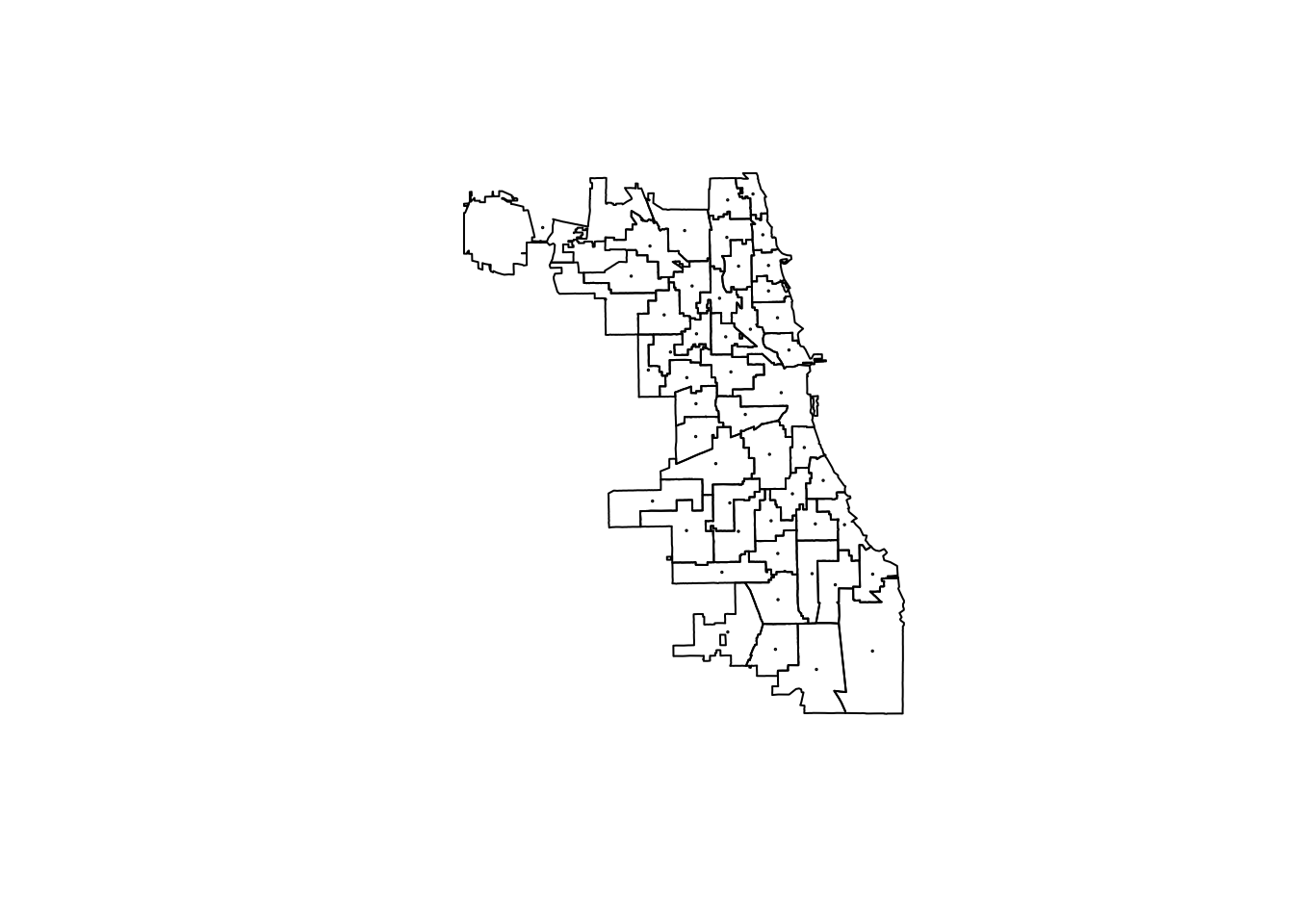# Chapter 2 Single-Dataset GIS Operations

## 2.1 Learning Objectives

• Become familiar with several common single-dataset GIS operations
• Calculate centroids of polygons
• Create buffers
• Explore additional single-dataset GIS operations

## 2.2 Functions Learned

• st_geometry()
• st_centroid()
• st_buffer()
• st_coordinates()
• st_bbox()

## 2.3 Interactive Tutorial

This workshop’s script can be found here.

## 2.4 Exercises

• Project 1986 ward data into correct UTM projection
library(sf)
ward86 <- st_read("data/ward1986.shp")
## Reading layer ward1986' from data source /Users/angela/Desktop/Spatial Data Science/workshop-notes/data/ward1986.shp' using driver ESRI Shapefile'
## Simple feature collection with 51 features and 1 field (with 1 geometry empty)
## geometry type:  MULTIPOLYGON
## dimension:      XY
## bbox:           xmin: -87.9402 ymin: 41.6443 xmax: -87.524 ymax: 42.0231
## epsg (SRID):    4269
## proj4string:    +proj=longlat +ellps=GRS80 +towgs84=0,0,0,0,0,0,0 +no_defs
ward86 <- st_transform(ward86, 32616)
plot(ward86)• Calculate centroids of wards with st_centroid
?st_centroid

centroids <- st_centroid(ward86)
## Warning in st_centroid.sf(ward86): st_centroid assumes attributes are
## constant over geometries of x
plot(st_geometry(centroids), cex = 0.1)
plot(st_geometry(ward86), add = T)plot(st_geometry(ward86))
plot(st_geometry(centroids), cex = 0.1, add = T)• Calculate bounding box with st_bbox`
• Plot centroids, buffered centroids, and wards for each year